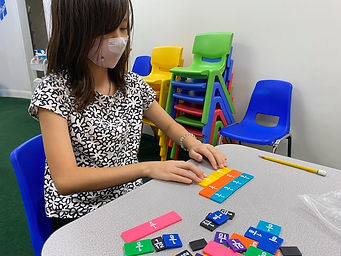## Ms. Dawn

### Target 1​

###### Lesson Type:

Continuation

Number Operation

:

Fractional Numbers

Convert between fractions, decimals, and percentages.

###### 1:

Convert fractions to decimals and decimals to fractions.

###### 2:

Understand that the digit to the left of the decimal is the whole number, the digits to the right are the numerator, and the denominator is the place-value of the furthest right number.

4th

###### Vocabulary:

Convert, Fractions, Tenths, Hundredths, Thousandths, Place Value, Decimal, Digit, Model

Activities:

1. Students reviewed place value with fractions (10,100, 1000).

2. Students turned fractions into decimals and decimals into fractions.

3. Students played a game of "Musical Fractions and Decimals." Students needed to pass their paper to the left after a certain amount of time whether they finished the problems on the sheet or not. The objective was to turn fractions and mixed numbers into decimals.

4. Students were given models of tenths and hundredths. They needed to figure out the fraction, decimal, and word form.### Home Exploration

###### Guiding Questions:## Absent Students:

### Target 2

:

###### 1:

Understand that fractions represent quantities that have a portion that is less than a whole.

###### 2:

Understand that fractions with different numerators and denominators can represent the same size.

4th

###### Vocabulary:

Equivalent Fraction, Quantities, Portion, Numerator, Denominator, Fraction Bars, Pie Fractions, Shaded

Activities:

1. To introduce equivalent fractions, students were given a fraction card. Then, they used fraction manipulative bars to show the given fraction and then show other equivalent fractions with other fraction bars. For example, if they were given 4/8, they showed 4/8 with the bars first. Then, they used other fraction bars to show what was equivalent to 4/8. For example, they laid out 2/4 and 1/2.

2. Students were given pie fractions that were shaded. They needed to figure out what each pie fraction represented and find the equivalent fraction based on the other pie fraction.

3. After practice with pictures on both ends, students were given only one side. They needed to figure out an equivalent fraction and shade it and state what that fraction represents.

4. Next, students were given fractions and on the other side either a numerator or denominator. They needed to figure out the missing number based on equivalent fractions rules.

5. Lastly, I gave them a fraction and they had to write down a few equivalent fractions.### Home Exploration

###### Guiding Questions:### Target 3

:

###### Vocabulary:

Activities:### Home Exploration